## Main.ControlTypes History

December 08, 2019, at 09:15 AM by 216.129.163.16 -
Changed line 15 from:

Objective: Design a model predictive controller for an overhead crane. Meet specific control objectives by tuning the controller and using the state space model of the crane system. Simulate and optimize the pendulum system with an adjustable overhead cart. Estimated time: 2 hours.

to:

Objective: Design a model predictive controller for an overhead crane with a pendulum mass. Meet specific control objectives by tuning the controller and using the state space model of the crane system. Simulate and optimize the pendulum system with an adjustable overhead cart. Estimated time: 2 hours.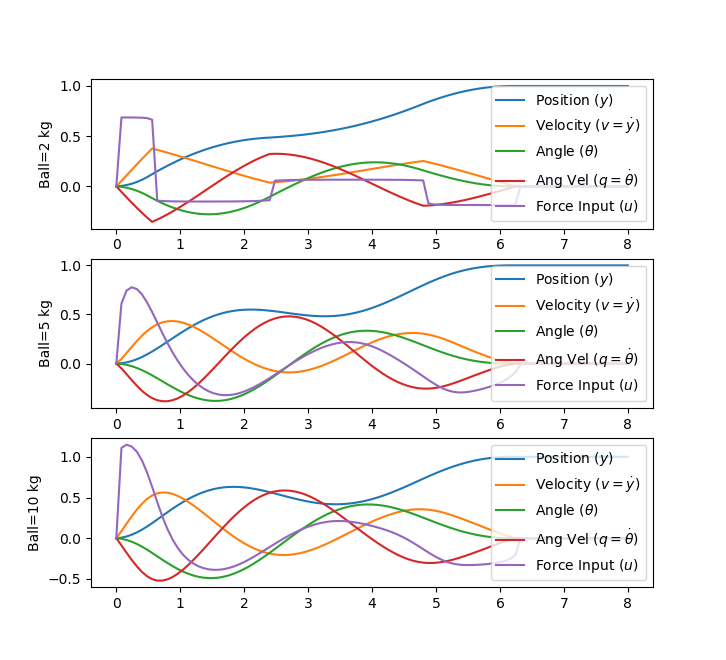(:divend:)

(:toggle hide gekko_ss button show="Show GEKKO (Python) State Space Model and Controller":) (:div id=gekko_ss:) (:source lang=python:) import numpy as np from gekko import GEKKO import matplotlib.pyplot as plt

mass = [2,5,10] nm = len(mass) plt.figure()

for j in range(len(mass)):

    m1 = 10
m2 = mass[j]
eps = m2/(m1+m2)
A = np.array([[0,1,0,0],
[0,0,eps,0],
[0,0,0,1],
[0,0,-1,0]])
B = np.array([,
,
,
[-1]])
C = np.identity(4)

m = GEKKO()
x,y,u = m.state_space(A,B,C,D=None)
m.time = np.linspace(0,8,101)
fn = [0 if m.time[i]<6.2 else 1 for i in range(101)]
final = m.Param(value=fn)
u.status = 1
m.Obj(final * ((y-1)**2 + y**2 + y**2 + y**2))
m.options.IMODE = 6
m.solve()

plt.subplot(len(mass),1,j+1)
plt.ylabel(f'Ball={mass[j]} kg')
plt.plot(m.time,y, label = r"Position ($y$)")
plt.plot(m.time,y, label = r"Velocity ($v=\dot y$)")
plt.plot(m.time,y, label = r"Angle ($\theta$)")
plt.plot(m.time,y, label = r"Ang Vel ($q=\dot \theta$)")
plt.plot(m.time,u, label = r"Force Input ($u$)")
plt.legend(loc=1)


plt.show() (:sourceend:)

Thanks to Derek Prestwich for the state space implementation.

April 02, 2018, at 10:06 PM by 10.37.28.47 -
March 22, 2018, at 12:20 AM by 10.37.35.33 -
Changed line 19 from: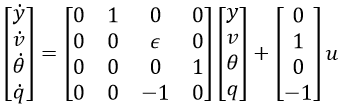to:

$$\begin{bmatrix} \dot y \\ \dot v \\ \dot \theta \\ \dot q \end{bmatrix}=\begin{bmatrix} 0 & 1 & 0 & 0\\ 0 & 0 & \epsilon & 0\\ 0 & 0 & 0 & 1\\ 0 & 0 & -1 & 0 \end{bmatrix} \begin{bmatrix} y \\ v \\ \theta \\ q \end{bmatrix} + \begin{bmatrix} 0 \\ 1 \\ 0 \\ -1 \end{bmatrix} u$$

March 04, 2018, at 06:12 AM by 45.56.3.173 -

(:html:) <iframe width="560" height="315" src="https://www.youtube.com/embed/clMBeJ3FI_g" frameborder="0" allow="autoplay; encrypted-media" allowfullscreen></iframe> (:htmlend:)

March 04, 2018, at 05:32 AM by 45.56.3.173 -
Changed line 21 from:

where m1=10 is the mass of the cart, m2=1 is the mass of the item carried, epsilon is m2/(m1+m2), y is the position of the overhead cart, v is the velocity of the overhead cart, theta is the angle of the pendulum relative to the cart, and q is the rate of angle change2.

to:

where m1=10 is the mass of the cart, m2=1 is the mass of the item carried, \epsilon is m2/(m1+m2), y is the position of the overhead cart, v is the velocity of the overhead cart, \theta is the angle of the pendulum relative to the cart, and q is the rate of angle change2.

March 04, 2018, at 05:10 AM by 45.56.3.173 -
Changed lines 227-228 from:

to:

Changed lines 235-237 from:

mass1, = ax.plot([],[],linestyle='None',marker='s',markersize=40,markeredgecolor='k',color='orange',markeredgewidth=2) mass2, = ax.plot([],[],linestyle='None',marker='o',markersize=20,markeredgecolor='k',color='orange',markeredgewidth=2) line, = ax.plot([],[],'o-',color='orange',lw=4,markersize=6,markeredgecolor='k',markerfacecolor='k')

to:

mass1, = ax.plot([],[],linestyle='None',marker='s', markersize=40,markeredgecolor='k', color='orange',markeredgewidth=2) mass2, = ax.plot([],[],linestyle='None',marker='o', markersize=20,markeredgecolor='k', color='orange',markeredgewidth=2) line, = ax.plot([],[],'o-',color='orange',lw=4, markersize=6,markeredgecolor='k', markerfacecolor='k')

Changed lines 263-265 from:

ani_a = animation.FuncAnimation(fig, animate, np.arange(1,len(m.time)),

                              interval=40,blit=False,init_func=init)

to:

ani_a = animation.FuncAnimation(fig, animate, np.arange(1,len(m.time)), interval=40,blit=False,init_func=init)

Changed line 272 from:

Thanks to https://www.linkedin.com/in/everton-colling-0161b047/? for the animation code in Python.

to:

Thanks to Everton Colling for the animation code in Python.

March 04, 2018, at 05:06 AM by 45.56.3.173 -
Deleted lines 36-41:Crane Pendulum Solution Files in MATLAB and Python

(:html:) <iframe width="560" height="315" src="https://www.youtube.com/embed/iTaz8ro-4D4" frameborder="0" allowfullscreen></iframe> (:htmlend:)Crane Pendulum Solution Files in MATLAB and Python

(:html:) <iframe width="560" height="315" src="https://www.youtube.com/embed/iTaz8ro-4D4" frameborder="0" allowfullscreen></iframe> (:htmlend:)

March 04, 2018, at 05:05 AM by 45.56.3.173 -

(:toggle hide gekko_animate button show="Show GEKKO (Python) and Animation Code":) (:div id=gekko_animate:) (:source lang=python:)

1. Contributed by Everton Colling

import matplotlib.animation as animation import numpy as np from gekko import GEKKO

1. requires ffmpeg to save mp4 file
2. available from https://ffmpeg.zeranoe.com/builds/
3. add ffmpeg.exe to path such as C:\ffmpeg\bin\ in
4. environment variables
5. Defining a model

m = GEKKO()

1. Weight of item

m2 = 5

1. Defining the time, we will go beyond the 6.2s
2. to check if the objective was achieved

m.time = np.linspace(0,8,100)

1. Parameters

m1a = m.Param(value=10) m2a = m.Param(value=m2) final = np.zeros(len(m.time)) for i in range(len(m.time)):

    if m.time[i] < 6.2:
final[i] = 0
else:
final[i] = 1


final = m.Param(value=final)

1. MV

ua = m.Var(value=0)

1. State Variables

theta_a = m.Var(value=0) qa = m.Var(value=0) ya = m.Var(value=-1) va = m.Var(value=0)

1. Intermediates

epsilon = m.Intermediate(m2a/(m1a+m2a))

1. Defining the State Space Model

m.Equation(ya.dt() == va) m.Equation(va.dt() == epsilon*theta_a + ua) m.Equation(theta_a.dt() == qa) m.Equation(qa.dt() == -theta_a -ua)

1. Definine the Objectives
2. Make all the state variables be zero at time >= 6.2

m.Obj(final*ya**2) m.Obj(final*va**2) m.Obj(final*theta_a**2) m.Obj(final*qa**2)

1. Try to minimize change of MV over all horizon

m.Obj(0.001*ua**2)

m.options.IMODE = 6 #MPC m.solve() #(disp=False)

1. Plotting the results

import matplotlib.pyplot as plt plt.figure(figsize=(12,10))

plt.subplot(221) plt.plot(m.time,ua.value,'m',lw=2) plt.legend([r'$u$'],loc=1) plt.ylabel('Force') plt.xlabel('Time') plt.xlim(m.time,m.time[-1])

plt.subplot(222) plt.plot(m.time,va.value,'g',lw=2) plt.legend([r'$v$'],loc=1) plt.ylabel('Velocity') plt.xlabel('Time') plt.xlim(m.time,m.time[-1])

plt.subplot(223) plt.plot(m.time,ya.value,'r',lw=2) plt.legend([r'$y$'],loc=1) plt.ylabel('Position') plt.xlabel('Time') plt.xlim(m.time,m.time[-1])

plt.subplot(224) plt.plot(m.time,theta_a.value,'y',lw=2) plt.plot(m.time,qa.value,'c',lw=2) plt.legend([r'$\theta$',r'$q$'],loc=1) plt.ylabel('Angle') plt.xlabel('Time') plt.xlim(m.time,m.time[-1])

plt.rcParams['animation.html'] = 'html5'

x1 = ya.value y1 = np.zeros(len(m.time))

1. suppose that l = 1

x2 = 1*np.sin(theta_a.value)+x1 x2b = 1.05*np.sin(theta_a.value)+x1 y2 = -1*np.cos(theta_a.value)+y1 y2b = -1.05*np.cos(theta_a.value)+y1

fig = plt.figure(figsize=(8,6.4)) ax = fig.add_subplot(111,autoscale_on=False,xlim=(-1.5,0.5),ylim=(-1.2,0.4)) ax.set_xlabel('position') ax.get_yaxis().set_visible(False)

crane_rail, = ax.plot([-1.5,0.5],[0.2,0.2],'k-',lw=4) start, = ax.plot([-1,-1],[-1.5,1],'k:',lw=2) objective, = ax.plot([0,0],[-1.5,1],'k:',lw=2) mass1, = ax.plot([],[],linestyle='None',marker='s',markersize=40,markeredgecolor='k',color='orange',markeredgewidth=2) mass2, = ax.plot([],[],linestyle='None',marker='o',markersize=20,markeredgecolor='k',color='orange',markeredgewidth=2) line, = ax.plot([],[],'o-',color='orange',lw=4,markersize=6,markeredgecolor='k',markerfacecolor='k') time_template = 'time = %.1fs' time_text = ax.text(0.05,0.9,'',transform=ax.transAxes) start_text = ax.text(-1.06,-1.1,'start',ha='right') end_text = ax.text(0.06,-1.1,'objective',ha='left')

def init():

    mass1.set_data([],[])
mass2.set_data([],[])
line.set_data([],[])
time_text.set_text('')
return line, mass1, mass2, time_text


def animate(i):

    mass1.set_data([x1[i]],[y1[i]+0.1])
mass2.set_data([x2b[i]],[y2b[i]])
line.set_data([x1[i],x2[i]],[y1[i],y2[i]])
time_text.set_text(time_template % m.time[i])
return line, mass1, mass2, time_text


ani_a = animation.FuncAnimation(fig, animate, np.arange(1,len(m.time)),

                              interval=40,blit=False,init_func=init)


ani_a.save('Pendulum_Control.mp4',fps=30)

plt.show() (:sourceend:)

Thanks to https://www.linkedin.com/in/everton-colling-0161b047/? for the animation code in Python.

(:divend:)

March 04, 2018, at 05:01 AM by 45.56.3.173 -
Changed lines 31-32 from:
to: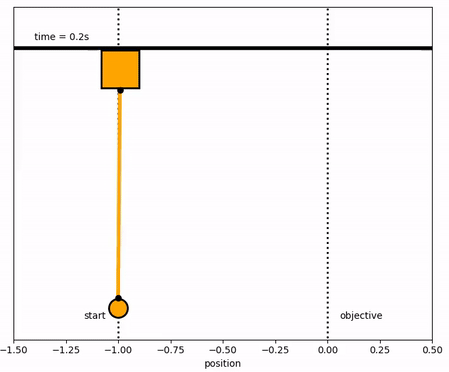Changed line 35 from:
to:March 04, 2018, at 05:00 AM by 45.56.3.173 -
Changed lines 31-32 from:to:
Changed line 35 from:to:
March 04, 2018, at 04:08 AM by 45.56.3.173 -
Changed line 33 from:

Solution with m2=1

to:

Solution with m2=5

March 04, 2018, at 04:07 AM by 45.56.3.173 -

Solution with m2=1

Solution with m2=1March 04, 2018, at 04:05 AM by 45.56.3.173 -Changed line 15 from:

Objective: Design a model predictive controller with a custom objective function that satisfies a specific problem criterion. Simulate and optimize a pendulum system with an adjustable overhead cart. Estimated time: 3 hours.

to:

Objective: Design a model predictive controller for an overhead crane. Meet specific control objectives by tuning the controller and using the state space model of the crane system. Simulate and optimize the pendulum system with an adjustable overhead cart. Estimated time: 2 hours.

Changed lines 92-107 from:

plt.subplot(5,1,1) plt.plot(m.time,u.value) plt.ylabel('u') plt.subplot(5,1,2) plt.plot(m.time,v.value) plt.ylabel('velocity') plt.subplot(5,1,3) plt.plot(m.time,y.value) plt.ylabel('y') plt.subplot(5,1,4) plt.plot(m.time,theta.value) plt.ylabel('theta') plt.subplot(5,1,5) plt.plot(m.time,q.value) plt.ylabel('q') plt.xlabel('time')

to:

plt.subplot(4,1,1) plt.plot(m.time,u.value,'r-',LineWidth=2) plt.ylabel('Force') plt.legend(['u'],loc='best') plt.subplot(4,1,2) plt.plot(m.time,v.value,'b--',LineWidth=2) plt.legend(['v'],loc='best') plt.ylabel('Velocity') plt.subplot(4,1,3) plt.plot(m.time,y.value,'g:',LineWidth=2) plt.legend(['y'],loc='best') plt.ylabel('Position') plt.subplot(4,1,4) plt.plot(m.time,theta.value,'m-',LineWidth=2) plt.plot(m.time,q.value,'k.-',LineWidth=2) plt.legend([r'$\theta$','q'],loc='best') plt.ylabel('Angle') plt.xlabel('Time')

(:toggle hide gekko button show="Show GEKKO (Python) Code":) (:div id=gekko:) (:source lang=python:)

1. Import packages

import numpy as np from gekko import GEKKO import matplotlib.pyplot as plt

1. Build model
2. initialize GEKKO model

m = GEKKO()

1. time

m.time = np.linspace(0,7,71)

1. Parameters

mass1 = m.Param(value=10) mass2 = m.Param(value=1) final = np.zeros(np.size(m.time)) for i in range(np.size(m.time)):

    if m.time[i] >= 6.2:
final[i] = 1
else:
final[i] = 0


final = m.Param(value=final)

1. Manipulated variable

u = m.Var(value=0)

1. Variables

theta = m.Var(value=0) q = m.Var(value=0)

1. Controlled Variable

y = m.Var(value=-1) v = m.Var(value=0)

1. Equations

m.Equations([y.dt() == v,

             v.dt() == mass2/(mass1+mass2) * theta + u,
theta.dt() == q,
q.dt() == -theta - u])

1. Objective

m.Obj(final * (y**2 + v**2 + theta**2 + q**2)) m.Obj(0.001 * u**2)

1. Tuning
2. global

m.options.IMODE = 6 #control

1. Solve

m.solve()

1. Plot solution

plt.figure() plt.subplot(5,1,1) plt.plot(m.time,u.value) plt.ylabel('u') plt.subplot(5,1,2) plt.plot(m.time,v.value) plt.ylabel('velocity') plt.subplot(5,1,3) plt.plot(m.time,y.value) plt.ylabel('y') plt.subplot(5,1,4) plt.plot(m.time,theta.value) plt.ylabel('theta') plt.subplot(5,1,5) plt.plot(m.time,q.value) plt.ylabel('q') plt.xlabel('time') plt.show() (:sourceend:) (:divend:)

March 10, 2017, at 04:04 PM by 10.5.113.121 -
Changed line 1 from:

(:title Control Basics: PID, LQR, MPC:)

to:

(:title Model Predictive Control:)

February 27, 2017, at 06:45 PM by 174.148.206.180 -
Deleted lines 20-23:
  m1 = 10
m2 = 1

Changed line 25 from:

The objective of the controller is to adjust the force on the cart to move the pendulum mass to a new final position. Ensure that initial and final velocities and angles of the pendulum are zero. The position of the pendulum mass is initially at -1 and it is desired to move it to the new position of 0 within 6.2 seconds. Demonstrate controller performance with changes in the pendulum position and that the final pendulum mass remains at the final position without oscillation.

to:

The objective of the controller is to adjust the force on the cart to move the pendulum mass to a new final position. Ensure that initial and final velocities and angles of the pendulum are zero. The position of the pendulum mass is initially at -1 and it is desired to move it to the new position of 0 within 6.2 seconds. Demonstrate controller performance with changes in the pendulum position and that the final pendulum mass remains at the final position without oscillation. How does the solution change if the mass of the item carried is increased to m2=5?

February 27, 2017, at 06:44 PM by 174.148.206.180 -
Changed lines 21-25 from:

where epsilon is m2/(m1+m2), y is the position of the overhead cart, v is the velocity of the overhead cart, theta is the angle of the pendulum relative to the cart, and q is the rate of angle change2.

to:
  m1 = 10
m2 = 1


where m1=10 is the mass of the cart, m2=1 is the mass of the item carried, epsilon is m2/(m1+m2), y is the position of the overhead cart, v is the velocity of the overhead cart, theta is the angle of the pendulum relative to the cart, and q is the rate of angle change2.

May 19, 2015, at 05:50 AM by 174.148.96.66 -

(:html:) <iframe width="560" height="315" src="https://www.youtube.com/embed/iTaz8ro-4D4" frameborder="0" allowfullscreen></iframe> (:htmlend:)

May 18, 2015, at 03:27 PM by 45.56.3.184 -
Changed lines 5-11 from:

There are many methods to implement control including basic strategies such as a proportional-integral-derivative (PID) controller or more advanced methods such as model predictive techniques. The purpose of this section is to provide a tutorial overview of potential strategies for control of nonlinear systems with linear models. A following section relates methods to implement dynamic control with nonlinear models.

to:

There are many methods to implement control including basic strategies such as a proportional-integral-derivative (PID) controller or more advanced methods such as model predictive techniques. The purpose of this section is to provide a tutorial overview of potential strategies for control of nonlinear systems with linear models.

(:html:) <iframe width="560" height="315" src="https://www.youtube.com/embed/YHAA-uXhI0E?rel=0" frameborder="0" allowfullscreen></iframe> (:htmlend:)

A following section relates methods to implement dynamic control with nonlinear models.

May 18, 2015, at 03:22 PM by 45.56.3.184 -

#### References

May 18, 2015, at 03:22 PM by 45.56.3.184 -
Changed lines 5-6 from:

There are many methods to implement control including basic strategies such as a proportional-integral-derivative (PID) controller or more advanced methods such as model predictive techniques. The purpose of this section is to provide a tutorial overview of potential strategies for control of nonlinear systems. A following section relates methods to implement dynamic control with nonlinear models and large-scale constrained optimizers.

to:

There are many methods to implement control including basic strategies such as a proportional-integral-derivative (PID) controller or more advanced methods such as model predictive techniques. The purpose of this section is to provide a tutorial overview of potential strategies for control of nonlinear systems with linear models. A following section relates methods to implement dynamic control with nonlinear models.

Changed lines 9-18 from:

Objective: Design a controller to maintain temperature of a chemical reactor. Develop 3 separate controllers (PID, Linear MPC, Nonlinear MPC) in Python or MATLAB/Simulink. Demonstrate controller performance with steps in the set point and disturbance changes. Estimated time: 3 hours.CSTR Source Files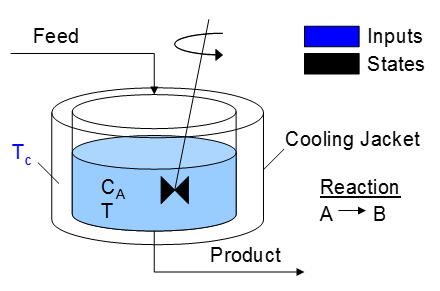A reactor is used to convert a hazardous chemical A to an acceptable chemical B in waste stream before entering a nearby lake. This particular reactor is dynamically modeled as a Continuously Stirred Tank Reactor (CSTR) with a simplified kinetic mechanism that describes the conversion of reactant A to product B with an irreversible and exothermic reaction. It is desired to maintain the temperature at a constant setpoint that maximizes the destruction of A (highest possible temperature).

to:

Objective: Design a model predictive controller with a custom objective function that satisfies a specific problem criterion. Simulate and optimize a pendulum system with an adjustable overhead cart. Estimated time: 3 hours.

A pendulum is described by the following dynamic equations:where epsilon is m2/(m1+m2), y is the position of the overhead cart, v is the velocity of the overhead cart, theta is the angle of the pendulum relative to the cart, and q is the rate of angle change2.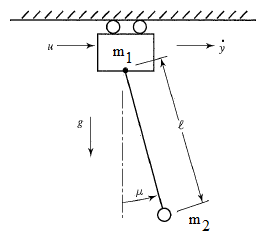The objective of the controller is to adjust the force on the cart to move the pendulum mass to a new final position. Ensure that initial and final velocities and angles of the pendulum are zero. The position of the pendulum mass is initially at -1 and it is desired to move it to the new position of 0 within 6.2 seconds. Demonstrate controller performance with changes in the pendulum position and that the final pendulum mass remains at the final position without oscillation.Crane Pendulum Solution Files in MATLAB and Python
1. Bryson, A.E., Dynamic Optimization, Addison-Wesley, 1999.
May 18, 2015, at 03:18 PM by 45.56.3.184 -
Changed line 9 from:

Objective: Design a model predictive controller to maintain temperature of a chemical reactor. Develop a linear, first-order model of the reactor and implement the controller in Python or MATLAB/Simulink. Demonstrate controller performance with steps in the set point and disturbance changes. Estimated time: 3 hours.

to:

Objective: Design a controller to maintain temperature of a chemical reactor. Develop 3 separate controllers (PID, Linear MPC, Nonlinear MPC) in Python or MATLAB/Simulink. Demonstrate controller performance with steps in the set point and disturbance changes. Estimated time: 3 hours.

May 13, 2015, at 06:06 PM by 10.5.113.160 -
May 09, 2015, at 12:30 AM by 10.5.113.160 -
Changed lines 9-13 from:

Objective: Design a model predictive controller to maintain temperature of a chemical reactor. Develop a linear, first-order model of the reactor and implement the model in Python or MATLAB/Simulink. Estimated time: 3 hours.

to:

Objective: Design a model predictive controller to maintain temperature of a chemical reactor. Develop a linear, first-order model of the reactor and implement the controller in Python or MATLAB/Simulink. Demonstrate controller performance with steps in the set point and disturbance changes. Estimated time: 3 hours.CSTR Source Files
May 09, 2015, at 12:26 AM by 10.5.113.160 -
Changed lines 5-16 from:

There are many methods to implement control including basic strategies such as a proportional-integral-derivative (PID) controller or more advanced methods such as model predictive techniques. The purpose of this section is to provide a tutorial overview of potential strategies for control of nonlinear systems. A following section relates methods to implement dynamic control with nonlinear models and large-scale constrained optimizers.

to:

There are many methods to implement control including basic strategies such as a proportional-integral-derivative (PID) controller or more advanced methods such as model predictive techniques. The purpose of this section is to provide a tutorial overview of potential strategies for control of nonlinear systems. A following section relates methods to implement dynamic control with nonlinear models and large-scale constrained optimizers.

#### Exercise

Objective: Design a model predictive controller to maintain temperature of a chemical reactor. Develop a linear, first-order model of the reactor and implement the model in Python or MATLAB/Simulink. Estimated time: 3 hours.A reactor is used to convert a hazardous chemical A to an acceptable chemical B in waste stream before entering a nearby lake. This particular reactor is dynamically modeled as a Continuously Stirred Tank Reactor (CSTR) with a simplified kinetic mechanism that describes the conversion of reactant A to product B with an irreversible and exothermic reaction. It is desired to maintain the temperature at a constant setpoint that maximizes the destruction of A (highest possible temperature).